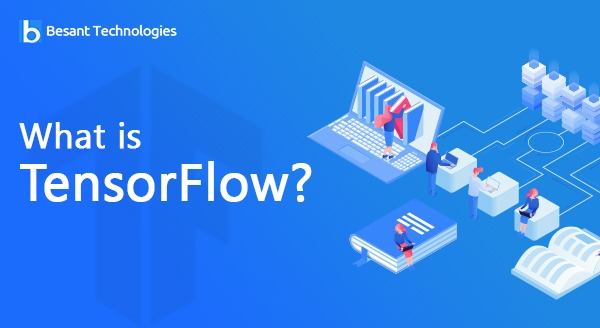Our Special Offer - Get 3 Courses at 24,999/- Only. Read more
Hire Talent (HR):+91-9707 240 250

# General# What is TensorFlow?

## TensorFlow

TensorFlow is a machine learning open library, known for its Python-friendly interface. This open-source library is used for numerical computations and can increase the rate at which machine learning can be achieved by the users. Formulated by Google, this product promises to evolve as one of the most successful deep learning libraries for virtual learners.

TensorFlow is a brainchild of Google’s research division and works at making large scale machine learning simpler. The product is designed keeping in view of researchers, programmers and data scientists. Using Python, TensorFlow helps provide a perfect combination of machine learning and neural networking models and makes it easier for the end-users.

### What is TensorFlow?

The popularity of deep learning as a ‘machine learning algorithm’ made Google use the neural networks to further enhance the experience of Gmail and search engine users. With this in mind, they developed their deep learning framework, TensorFlow which enabled the researchers and programmers to work on this model which works on AI.

Developed and put to use in the year 2015, a stable version of TensorFlow was not made available till 2017. Now, as a deep learning algorithm, it can be used by anyone, modified according to the requirement, and also redistribute amongst their users, without paying a hefty fee to Google for it.

### Where can we use TensorFlow?

TensorFlow can work in three sections

• For building a model
• For training and estimating the model created
• For data preprocessing

The name ‘TensorFlow’ comes from the fact that the inputs into the algorithm go as a multi-dimensional array, which is called Tensors. The users can create a ‘Graph’ or flowcharts of work or operations that need to be performed by the algorithm before it is feed. Now, once the input goes from one end, it passes through a series of systems of multiple operations to come out as the desired output.

Thus, TensorFlow aids the developers to create a Graph to describe how the data is supposed to move (Dataflow graphs) or processing nodes. Now, every node that the graph contains represents a ‘mathematical operation’, and the edge or the connection between each node denotes a multidimensional data array or is called a Tensor.

The data feeds and graphs can be fed in Python language, therefore, making it further easier for the programmers to work on their data. So, the nodes and tensors, as well as the TensorFlow, is completely operated upon as Python applications.

However, one needs to remember that the math operations on TensorFlow are performed through C++ binaries (high performance). What Python does is to direct the traffic between the pieces, and therefore, helps in providing a higher level of programming abstractions which can help in hooking the pieces together.

The model thus created can be run on different machines, once it is trained appropriately. Both GPU’s and CPUs can be used for training the models created using TensorFlow. Once implemented, the users can use the TensorBoard to monitor both graphically and visually, the progress of TensorFlow.

### What are the advantages of TensorFlow?

One of the main advantages of using TensorFlow is ‘Abstraction’. It allows the programmers to concentrate upon the logic of the application, instead of worrying about smaller things such as finding ways to attach the output of one function to the input of the other or even for implementing algorithms.

Also, the ‘eager execution’ model of TensorFlow helps in identifying and debugging the TensorFlow apps. This helps in the modification or evaluation of individual graphs separately. The TensorBoard again helps in inspecting the graphs.

Enroll Now!